• CN 62-1091/P
• ISSN 1001-8166
• 月刊 创刊于1986年

## 基于ICA的引潮力互相关谱分析

1. 昆明理工大学，信息工程与自动化学院,云南 昆明 650500

## Independent Component Extraction and Cross-correlation Spectrum Analysis of Gravity Tide Signal

1. Kunming University of Science and Technology, Faculty of Information Engineering and Automation, Kunming 650500, China

 基金资助: 国家自然科学基金项目“提取重力固体潮信号中地球物理信息和地震前兆信息的关键信号处理算法研究”.  编号：41364002

Received: 2018-09-13   Revised: 2018-12-08   Online: 2019-02-26Abstract

The gravity solid tide signal includes daily wave, half-day wave and annual wave and moon wave harmonic component, but the energy of day wave and half-day wave component is relatively strong, and the energy of annual wave and moon wave component is relatively weak. In order to effectively extract these harmonic components with large energy differences and reveal the modulation relationship between them, according to the cause of gravity tide, a gravity solid tide signal decomposition model is used to compare the tidal harmonic components with different strengths. The form of the independent component is decomposed into different orthogonal directions. At the same time, a new optimization algorithm is used to improve the independent component analysis algorithm and separate the independent components of different orthogonal directions. In the spectral correlation analysis of the components of independent components, the autocorrelation operation will make the strong component stronger and the weak component weaker. For this problem, the cross-correlation spectrum between independent components is used to reveal the gravity tide signal., the modulation relationship between harmonic components. The experimental results show that the proposed algorithm not only effectively separates the independent components with large intensity difference in the gravity tide signal from the perspective of additive decomposition, but also reveals the multiplicative modulation relationship between the corresponding tidal harmonics based on the cross-correlation spectrum.

Keywords： Gravity solid tide ; Intelligent optimization algorithm ; Independent Component Analysis ; Multiplicative demodulation ; Cross-correlation spectrum.

Xing Dezhao, Quan Haiyan. Independent Component Extraction and Cross-correlation Spectrum Analysis of Gravity Tide Signal. Advances in Earth Science[J], 2019, 34(1): 103-112 doi:10.11867/j.issn.1001-8166.2019.01.0103

## 2 重力固体潮正交分解模型

### 图1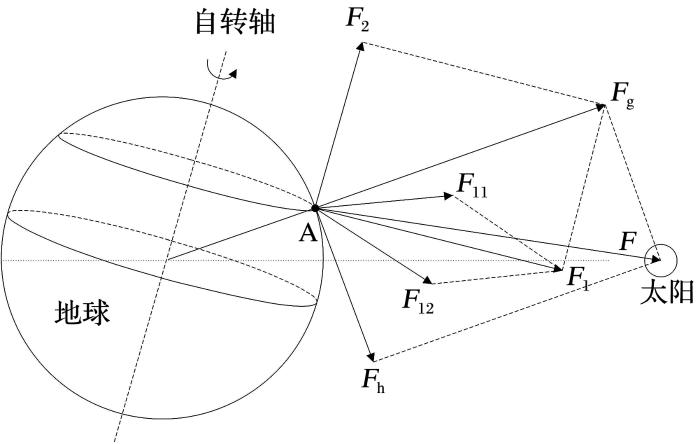Fig. 1   The orthogonal decomposition model of Gravity Earth Tide signal

## 3 基于一种新型智能优化算法的ICA

ICA是盲源信号的主要分析方法，基于独立性判据建立优化准则，并选择优化算法令目标函数趋于极大值或极小值的过程，使分离的独立分量最大限度地趋于原始信号[9,10]

2中，$S(t)$为观测到的混合信号$(s1,s2,⋯,sn)T$，通过去均值、白化得到$Z(t)$，再利用优化准则和优化算法得出解混矩阵$K$，通过$y(t)=K×Z(t)$，得到原始信号$y(t)$

### 图2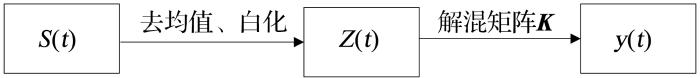Fig.2   Procedure of ICA

## 4 基于互相关的谱相关分析

${y1(t)}$为零均值的非平稳复信号，${y2(t)}$为另一路零均值的非平稳复信号，2路信号的互相关函数可表示为：

$Ry1y2(t,τ)=E[y1(t+τ/2)y2*(t-τ/2)]$

$Ry1y2(t,τ)$周期为$T0$,则：

$Ry1y2(t,τ)=limN→∞12N+1∑y1(t+nT0+τ/2)y2×(t+nT0-τ/2)$ ,

$Ry1y2(t,τ)=∑n=-∞∞Ry1y2a(τ)ej2πat$

$Ry1y2a(τ)=limT→∞1T∫-T/2T/2y1(t+τ/2)y2*(t-τ/2)e-j2πatdt=y1(t+τ/2)y2*(t-τ/2)e-j2πatt,$

$Sy1y2a(f)=∫-∞∞Ry1y2a(τ)e-j2πfτdτ$,

## 5 基于ICA的引潮力互相关谱分析

（1）获取观测点处的重力固体潮信号。为了便于计算，本文选取同一经度、不同纬度的3路重力固体潮信号。

（2）$①$对混合信号$S(t)$进行去均值、白化得到$Z(t)$$②$将粒子群的m个粒子初始化，即将每个粒子对应于一个正交的分离矩阵$K(0)$，并生成m个随机的正交分离矩阵。$③$利用每个粒子对应的正交的分离矩阵$K(c)$,进行粒子到邻域的搜索，得到最新的正交分离矩阵$K(c+1)$$④$利用最新获得的正交分离矩阵$K(c+1)$，实现白化信号$Z(t)$的变换，得到最新的分离信号$y(t)$$⑤$根据目标函数(优化准则)，求取各个粒子对应的最新的评价函数值，根据评价函数值，得到各个粒子不同角色态所对应的正交分离矩阵$K(c+1)$，记录最好粒子所对应的正交矩阵$K*$。若最好粒子所对应的评价函数值已经收敛至不变，则跳到步骤$⑥$，否则跳到步骤$③$，并进行下一次的搜索评价。$⑥Z(t)$再与解混矩阵$K*$进行运算，得到解混输出信号$y(t)$

（3）对解混输出信号$y(t)$做傅里叶变换，然后利用公式(4)和(5)对其进行处理，实现信号的乘性解调。

（4）读取重力固体潮信号中相应的物理信息，并结合潮汐谐波理论计算值进行对比分析（详见下文），进而求出各谐波分量间的调制关系。

### 图3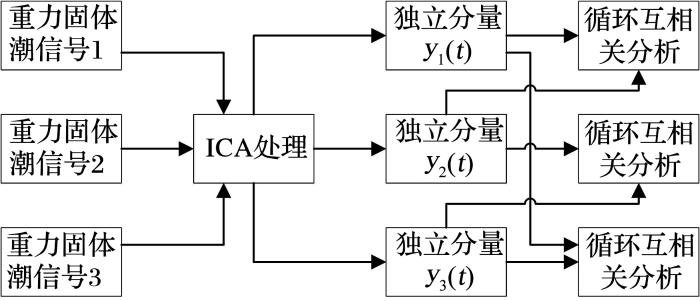Fig. 3   Flow chart of ICA and cross-correlation spectral analysis

## 6 实验结果分析

### 图4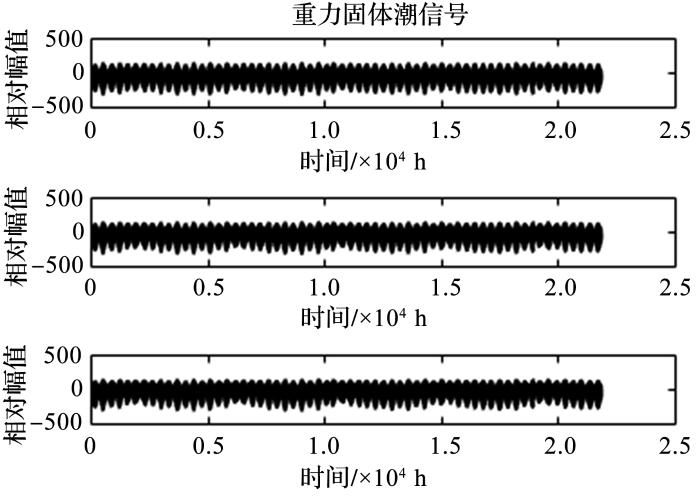Fig. 4   Gravity solid tidal signal of Kunming

### 图5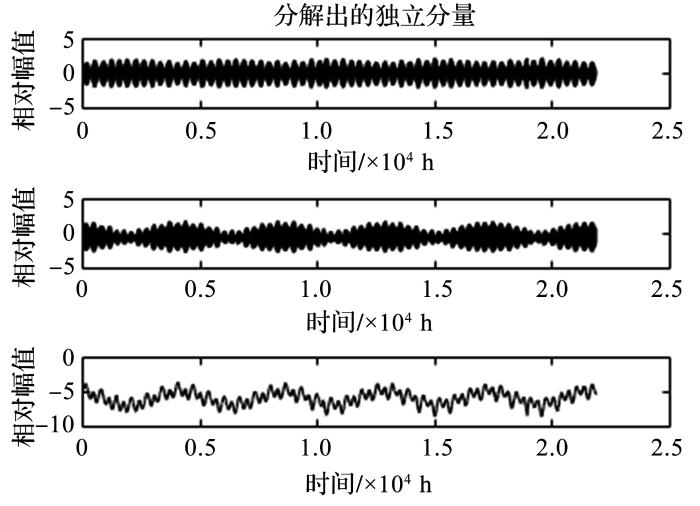Fig. 5   Waveform of independent component of ICA

### 图6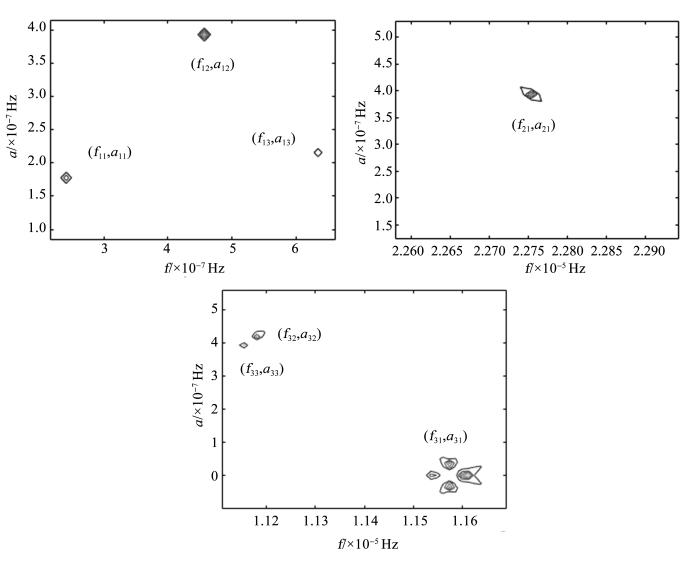Fig.6   Autocorrelation spectrum of y1(t),y2(t) and y3(t)

Table 1  Autocorrelation spectrum analysis of y1(t) ,y2(t) and y3(t)

$f11=2.411×10-7$

$a11=1.777×10-7$

$F1=f11+a11=4.188×10-7$$4.2004×10-7$
$F2=f11-a11=6.34×10-8$$6.3377×10-8$

$f12=4.569×10-7$

$a12=3.934×10-7$

$F3=f12+a12=8.503×10-7$$8.4725×10-7$
$F4=f12-a12=6.35×10-8$$6.3377×10-8$

$f13=6.345×10-7$

$a13=2.157×10-7$

$F5=f13+a13=8.502×10-7$$8.4725×10-7$
$F6=f13+a13=4.188×10-7$$4.2004×10-7$

$f21=2.275×10-5$

$a21=3.934×10-7$

$F7=f21+a21=2.3143×10-5$$2.3148×10-5$
$F8=f21-a21=2.2357×10-5$$2.2364×10-5$

$f31=1.157×10-5$

$a31=3.807×10-8$

$F9=f31+a31=1.1608×10-5$$1.1606×10-5$
$F10=f31-a31=1.1532×10-5$$1.1542×10-5$

$f32=1.118×10-5$

$a32=4.188×10-7$

$F11=f32+a32=1.1599×10-5$$1.1606×10-5$
$F12=f32-a32=1.0761×10-5$$1.0759×10-5$

$f33=1.115×10-5$

$a33=3.934×10-7$

$F13=f33+a33=1.1543×10-5$$1.1542×10-5$
$F14=f33-a33=1.0757×10-5$$1.0759×10-5$

### 图7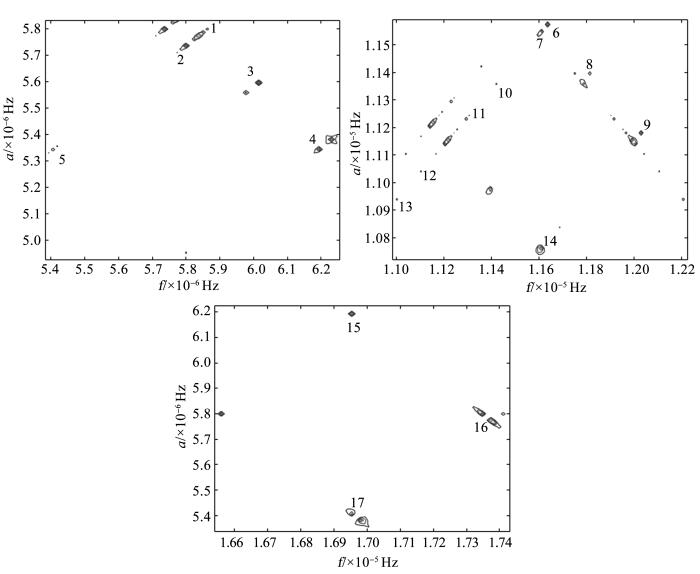Fig.7   Cross-correlation spectrum of y1(t),y2(t) and y3(t)

Table 2  Cross-correlation peaks of y1(t),y2(t) and y3(t)

1$(5.863×10-6,5.799×10-6)$2$(5.774×10-6,5.711×10-6)$3$(6.015×10-6,5.596×10-6)$
4$(6.193×10-6,5.343×10-6)$5$(5.406×10-6,5.343×10-6)$6$(1.164×10-5,1.157×10-5)$
7$(1.16×10-5,1.154×10-5)$8$(1.181×10-5,1.14×10-5)$9$(1.203×10-5,1.118×10-5)$
10$(1.142×10-5,1.136×10-5)$11$(1.131×10-5,1.124×10-5)$12$(1.11×10-5,1.104×10-5)$
13$(1.1×10-5,1.094×10-5)$14$(1.161×10-5,1.076×10-5)$15$(1.695×10-5,6.193×10-6)$
16$(1.737×10-5,5.774×10-6)$17$(1.695×10-5,5.406×10-6)$

Table 3  Cross correlation spectrum analysis of y1(t),y2(t) and y3(t)

$6.3377×10-8$1，2，5，6，7，10，11，12，13
$4.2004×10-7$3，8
$8.4725×10-7$4，9，14

$1.0759×10-5$14155
$1.1186×10-5$9
$1.1511×10-5$82
$1.1542×10-5$7174
$1.1574×10-5$6
$1.1606×10-5$7，14163
$1.1637×10-5$6
$1.1669×10-5$81
$1.2026×10-5$9
$1.2453×10-5$

$2.1580×10-5$
$2.1944×10-5$13
$2.2000×10-5$12
$2.2364×10-5$14，17
$2.2728×10-5$11
$2.2784×10-5$10
$2.3116×10-5$
$2.3148×10-5$7，15，16
$2.318×10-5$
$2.3212×10-5$6，8，9

### 图8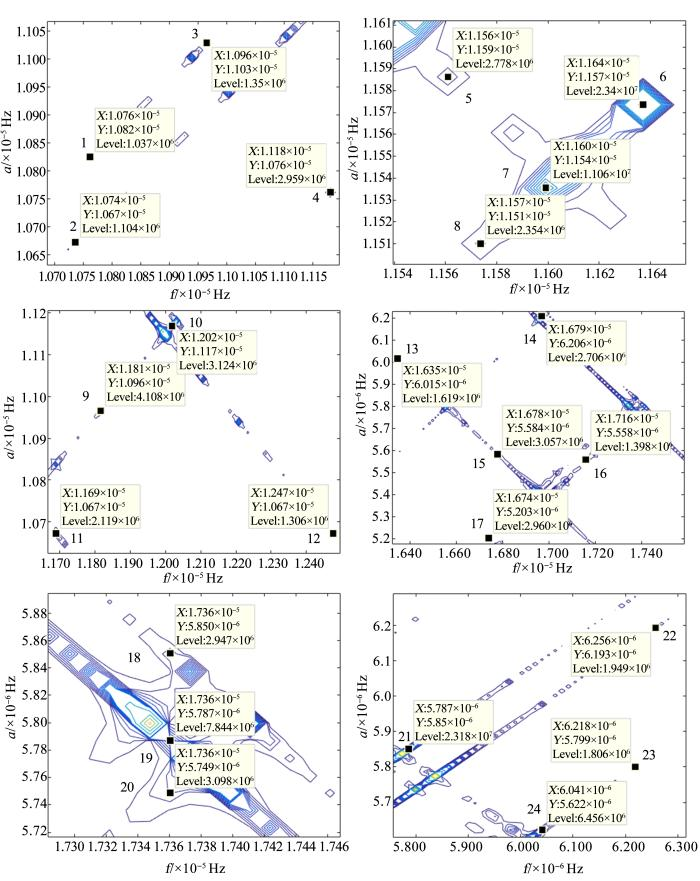Fig. 8   Partial cross-correlation spectrum of y1(t),y2(t) and y3(t)

Table 4  Partial cross-correlation spectral analysis of y1(t),y2(t) and y3(t)

$6.3377×10-8$1,2,3,21,22
$4.2004×10-7$23,24
$8.4725×10-7$9,

$1.0759×10-5$4,11,1214
$1.1186×10-5$4,1015
$1.1511×10-5$818
$1.1542×10-5$717
$1.1574×10-5$6,819
$1.1606×10-5$716,20
$1.1637×10-5$621
$1.1669×10-5$1124
$1.2026×10-5$1023
$1.2453×10-5$1222

$2.1580×10-5$1
$2.1944×10-5$17
$2.2000×10-5$3
$2.2364×10-5$13,15
$2.2728×10-5$16
$2.2784×10-5$9
$2.3116×10-5$20
$2.3148×10-5$5,19
$2.318×10-5$14
$2.3212×10-5$18

## 参考文献 原文顺序 文献年度倒序 文中引用次数倒序 被引期刊影响因子

Xu Houze .

Solid Earth Tide

[M]. Wuhan: Hubei Science and Technology Press, 2010.

[M].武汉: 湖北科学技术出版社2010.

Li Xingqiao , Zhang Chuanyin , Wang Wei , et al .

Analysis of influence of Earth tide on vertical deformation and gravity change in the Three Gorges area

[J]. Bulletin of Surveying and Mapping,2017, (3):9-12.

[J].测绘通报,2017, (3):9-12.

Zhang Chunguan , Yuan Bingqiang , Zhang Guoli .

Quality assessment of land gravity data in the latest global gravity database V23

[J]. Advances in Earth Science, 2017, 32 (1): 75-82.

[J].地球科学进展, 2017, 32(1): 75-82.

Quan Haiyan , Liu Yan .

Spectral correlation analysis of EMD modal components and demodulation analysis of gravity solid tide signals

EMD模态分量的谱相关分析法及其对重力固体潮信号的解调分析

[J].地球科学进展,2016,31(9):919-925.

Zhou Jiangcun , Sun Heping , Xu Jianqiao , et al .

Strain and stress tide in Earth

[J]. Chinese Journal of Geophysics, 2013,56(11):3 779-3 787.

[J].地球物理学报,2013,56(11):3 779-3 787.

Zhang Li , Fu Rongshan , Zhou Zhi , et al .

Seismic precursor information of gravity solid tides extracted by HHT

[J]. Acta Seismologica Sinica, 2007, 29(2): 222-226.

[J].地震学报, 2007, 29(2): 222-226.

Zhang Yan , Wu Yun , Liu Yongqi , et al .

Identification and extraction of seismic precursor information in tidal deformation data

[J].Journal of Geodesy and Geodynamics, 2003, 23(4): 34-39.

[J].大地测量与地球动力学, 2003, 23(4): 34-39.

Li Qiaoyan , Quan Haiyan .

The tidal harmonic extraction of gravity solid tide signal based on ICA

[J].Journal of Yunnan University (Natural Science Edition),2015,37(6):845-850.

[J].云南大学学报:自然科学版,2015,37(6):845-850.

Chen Hao , Bai Lin , Zhou Zhiyu .

Development and application of blind source separation technology

[J]. Space Electronic Technology, 2013, 10(1):5-10.

[J].空间电子技术，2013, 10(1):5-10.

Yu Xianchuan , Hu Dan .

Theory and Application of Blind Source Separation

[M]. Beijing: Science Press, 2011.

[M]. 北京科学出版社,2011.

Lee T , Girolami M Sejnowski T .

Independent component analysis using an extended infomax algorithm for mixed sub-Gaussian and super-Gaussian sources

[J]. Neural Computation, 1999, 11(2): 417- 441.

Zhang Xianda , Bao Zheng .

Blind signal separation

[J]. Acta Electronica Sinica,2001,(Suppl.1):1 766-1 771.

[J].电子学报，2001,(增刊 1):1 766-1 771.

Quan Haiyan , Shi Xinling .

A surface-simplex dwarm rvolution slgorithm

[J].Wuhan University Journal of Natural Sciences,2017,22(1):38-50.

Zhang Xianda , Bao Zheng .

Non-stationary Signal Analysis and Processing

[M]. Beijing: National Defense Industry Press, 1998.

[M].北京:国防工业出版社1998.

Huang Zhitao .

Cyclic and Stationary Signal Processing and Application

[M]. Beijing:Science Press,2006.

[M].北京科学出版社2006.

Lei Dian .

Non-stationary Signal Analysis Method Based on Cyclostationary Characteristics

[D]. Chengdu: University of Electronic Science and Technology, 2016.

[D].成都电子科技大学,2016.

Fang Jun .

Gravity and Solid Tide Course

[M]. Beijing: Seismological Press, 1982.

[M]. 北京地震出版社1982.

Xu Huajun , Liu Lintao , Luo Xiaowen .

Simulation implementation of global gravity solid tide

[J]. Journal of System Simulation,2009,21(24):7 824-7 827,7 832.

[J].系统仿真学报,2009,21(24):7 824-7 827,7 832.

Su Rong , Li Shengle .

The calculation of theoretical values of solid tide waves based on laplace series expansion method

[J]. Journal of Geodesy and Geodynamics,2013,33(5):106-109.

[J].大地测量与地球动力学,2013,33(5):106-109.

Gao Xiaoliang , Jing Lei , Sun Mingguo .

The calculation of theoretical value of gravity solid tide based on MATLAB

[J]. Western Science and Technology, 2009,8(1):33-34.

[J].中国西部科技,2009,8(1):33-34.

Wang Qingbin , Huang Guangping , Xu Peng .

The computer deduction of the tide level of solid tide

[J]. Journal of Institute of Surveying and Mapping, 2003, 20(2): 83-85.

[J].测绘学院学报, 2003, 20(2): 83-85.

Su Qihui , Duan Yanfei , Hu Yili , et al .

The closed formula of the theoretical derivative of gravity tides

[J]. Chinese Journal of Geophysics, 1994, 37(5): 647-658.

[J].地球物理学报, 1994, 37(5): 647-658.

Wu Qingpeng .

Calculation of the theoretical value of solid tide in a rotating elastic ellipsoidal earth model

[J]. Acta Seismologica Sinica,1990,12(3):282-291,337.

[J].地震学报,1990,12(3):282-291,337.

/

 〈〉